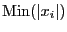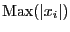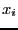Next: Specificity for the analysis Up: Parametric polynomial Previous: Possible parameters values for   Contents

## Condition number

The condition number of a polynomial may be defined either as the ratio lowest root over largest root or as the ratiooverwhereare the roots of the polynomial. In the later case the condition number has a value between 0 and 1. The minimal and maximal values of the condition number of a parametric polynomial in both form may be calculated using the procedure: